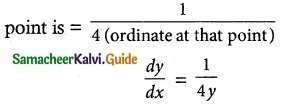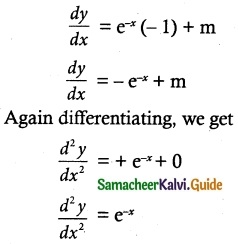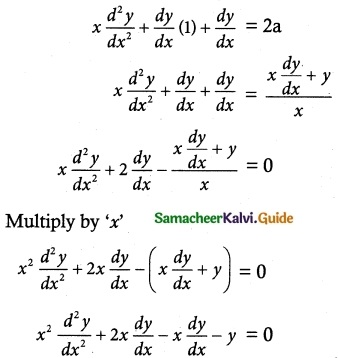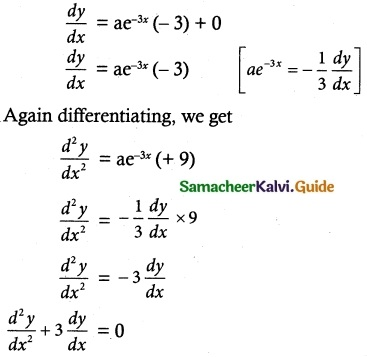Tamilnadu State Board New Syllabus Samacheer Kalvi 12th Maths Guide Pdf Chapter 10 Ordinary Differential Equations Ex 10.4 Textbook Questions and Answers, Notes.

## Tamilnadu Samacheer Kalvi 12th Maths Solutions Chapter 10 Ordinary Differential Equations Ex 10.4

Question 1.
Show that each of the following expressions is a solution of the corresponding given differential equation.
(i) y = 2x² ; xy’ = 2y
(ii) y = aex + be-x; y” – y = 0
Solution:
(i) v = 2x2 …(1)
Differential equation: xy’ = 2y
Differentiate with respect to ‘x’On simplifying, 2y = xy’
∴ (1) is solution of the given differential equation.(ii) y = aex + be-x; y”- y = 0.
y = aex + be-x …(1) Differential equation: y” – y = 0
Differentiate with respect to ‘x’
y’ = aex – be-x
Again differentiate with respect to ‘x’
y” = aex + be-x
y” = y ⇒ y” – y = 0
∴ (1) is the solution of the given differential equation.Question 2.
Find the value of m so that the function y = em solution of the given differential equation.
(i) y’ + 2y = 0
(ii) y”- 5y’ + 6y = 0
Solution:
(i) y’ + 2y = 0 ……. (1)
Given y = emx ……….(2)
Differentiating equation (2) w.r.t x, we get∴ comparing equation (1) & (3),
we get m = -2

(ii) y” – 5y’ + 6y = 0 ……. (1)
Given y = emx ……… (2)
Differentiating equation (2) w.r.t ‘x’, we get
$$\frac { dy }{ dx }$$ = ex. m
Again differentiating w.r.t ‘x’, we get
$$\frac { d^2y }{ dx^2 }$$ = emx. m²
To find the value of m:
Given y” – 5y’ + 6y = 0 ∵ y = emxm² – 5m + 6 = 0
(m – 2) (m – 3) = 0
∴ m = 2, 3Question 3.
The Slope of the tangent to the curve at any point is the reciprocal of four times the ordinate at that point. The curve passes through (2, 5). Find the equation of the curve.
Solution:
The slope of the tangent to the curve at anyThe equation can be written as
4y dy = dx …….. (1)
Integrating equation (1) on both sides, we get
4∫ ydy = ∫ dx
4($$\frac { y^2 }{ 2 }$$) = x + c
2y² = x + c …….. (2)
Since the curve passes through at (2, 5), we get
2(5)² = 2 + c
50 = 2 + c
50 – 2 = c
48 = c
∴ Substituting the value of c in equation (2), we get
2y² = x + 48 is the required equation of the curve.Question 4.
Show that y = e-x + mx + n is a solution of the differential equation ex($$\frac { d^2y }{ dx^2 }$$) – 1 = 0
Given y = e-x + mx + n …….. (1)
Differentiating equation (1) w.r.t ‘x’, we getsubstituting the value of $$\frac { d^2y }{ dx^2 }$$ in the given differential equation, we get
ex($$\frac { d^2y }{ dx^2 }$$) – 1
= ex(e-x) – 1
= en-1 = 1 – 1 = 0
Hence y = e-x + mx + n is the solution of the given differential equation ex $$\frac { d^2y }{ dx^2 }$$ – 1 = 0Question 5.
Show that y = ax + $$\frac { b }{ x }$$, x ≠ 0 is a solution of the differential equation x²yⁿ + xy’ – y = 0.
Solution:
GivenAgain differentiating we getx² y” + 2xy’ – xy’ – y = 0
x²y” + xy’ – y = 0
∴ y = ax + $$\frac { b }{ x }$$ is a solution of the differential equation x²y” + xy’ – y = 0Question 6.
Show that y = ae-3x + b, where a and b are arbitrary constants, is a solution of the differential equation $$\frac { d^2y }{ dx^2 }$$ + 3 $$\frac { dy }{ dx }$$ = 0.
Solution:
Given y = ae-3x + b …… (1)
Differentiating equation (1) w.r.t ‘x’, we getTherefore, y = ae-3x + b is a solution of the given differential equation.Question 7.
Show that the differential equation representing the family of curves y² = 2a(x + a2/3), where a is a positive parameter, is (y² – 2xy$$\frac { dy }{ dx }$$)³ = 8(y$$\frac { dy }{ dx }$$)5
Solution:
Given y² = 2ax + 2a5/3 ……. (1)
Differentiating equation (1) w.r.t ‘x’ we getHence y² = 2ox + 2 a³ is a solution of the differential equation
(y² – 2xy$$\frac { dy }{ dx }$$)³ = 8(y$$\frac { dy }{ dx }$$)5Question 8.
Show that y = a cos bx is a solution of the! differential equation $$\frac { d^2y }{ dx^2 }$$ + b² y = 0.
Solution:
Given y = a cos bx ……. (1)
Differentiating equation (1) w.r.t ‘x’, we get
$$\frac { dy }{ dx }$$ = a(-sin bx)b
$$\frac { dy }{ dx }$$ = -ab sin bx
Again differentiating, we getTherefore, y = a cos bx is a solution of the differential equation $$\frac { d^2y }{ dx^2 }$$ + b² y = 0